## 分界面

### 二分类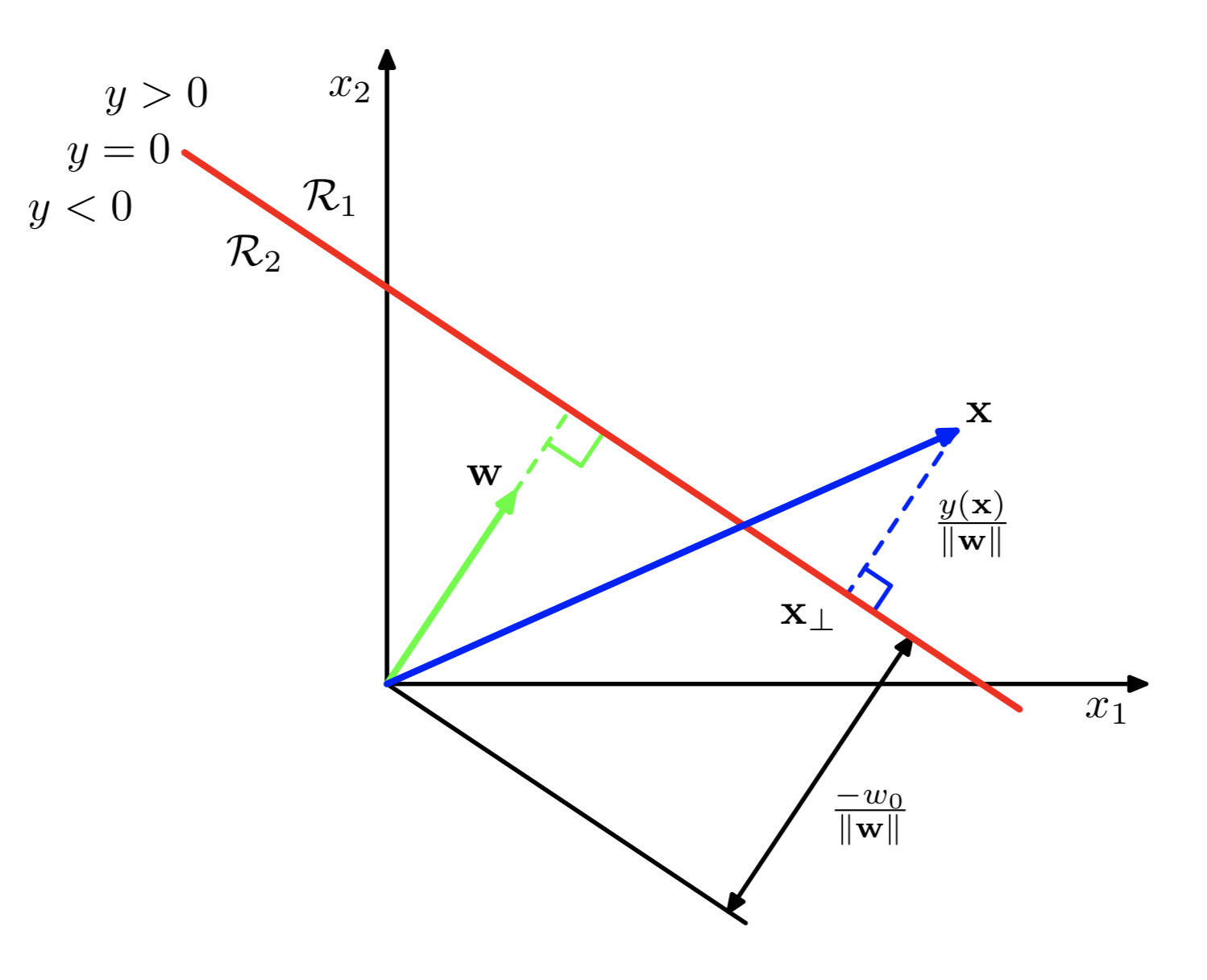### 多分类

1. One vs Rest：每类和剩下的所有类作分类，构建$K-1$个分类器
2. One vs One：每两类之间构建一个分类器，共$K(K-1)/2$
3. 构建K个分类器，类似于softmax，每个分类器输出一个得分，将样本预测为得分最高的那一类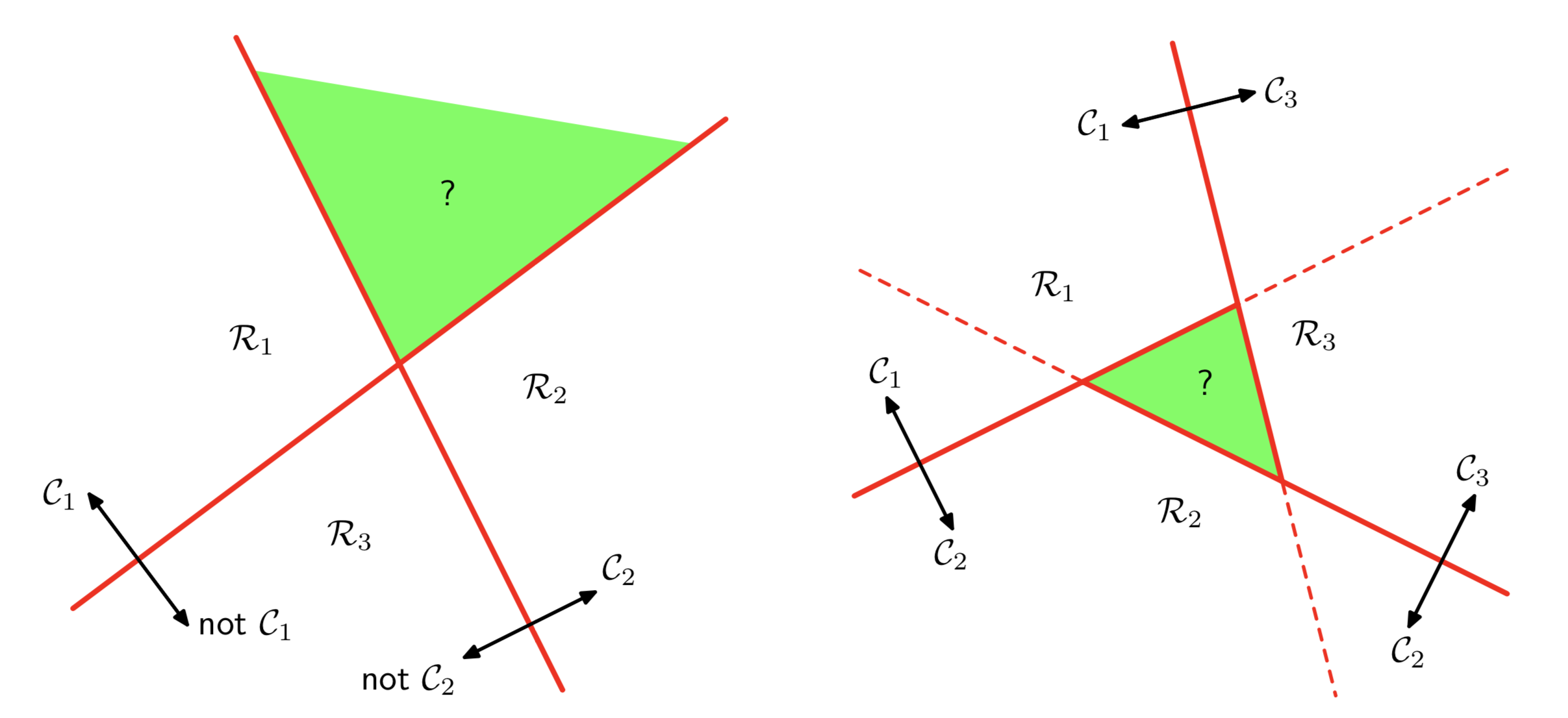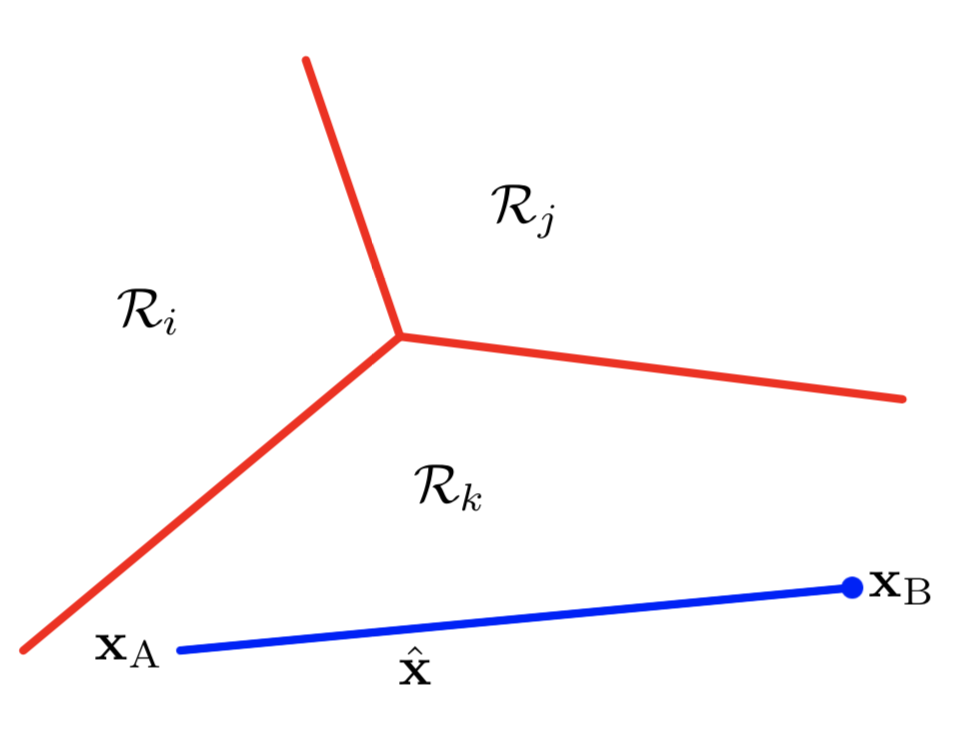## 几种分类方法

### Least squares

1. 对噪声敏感
2. 在分类问题上最小二乘法的假设不合理，在很多问题上会失败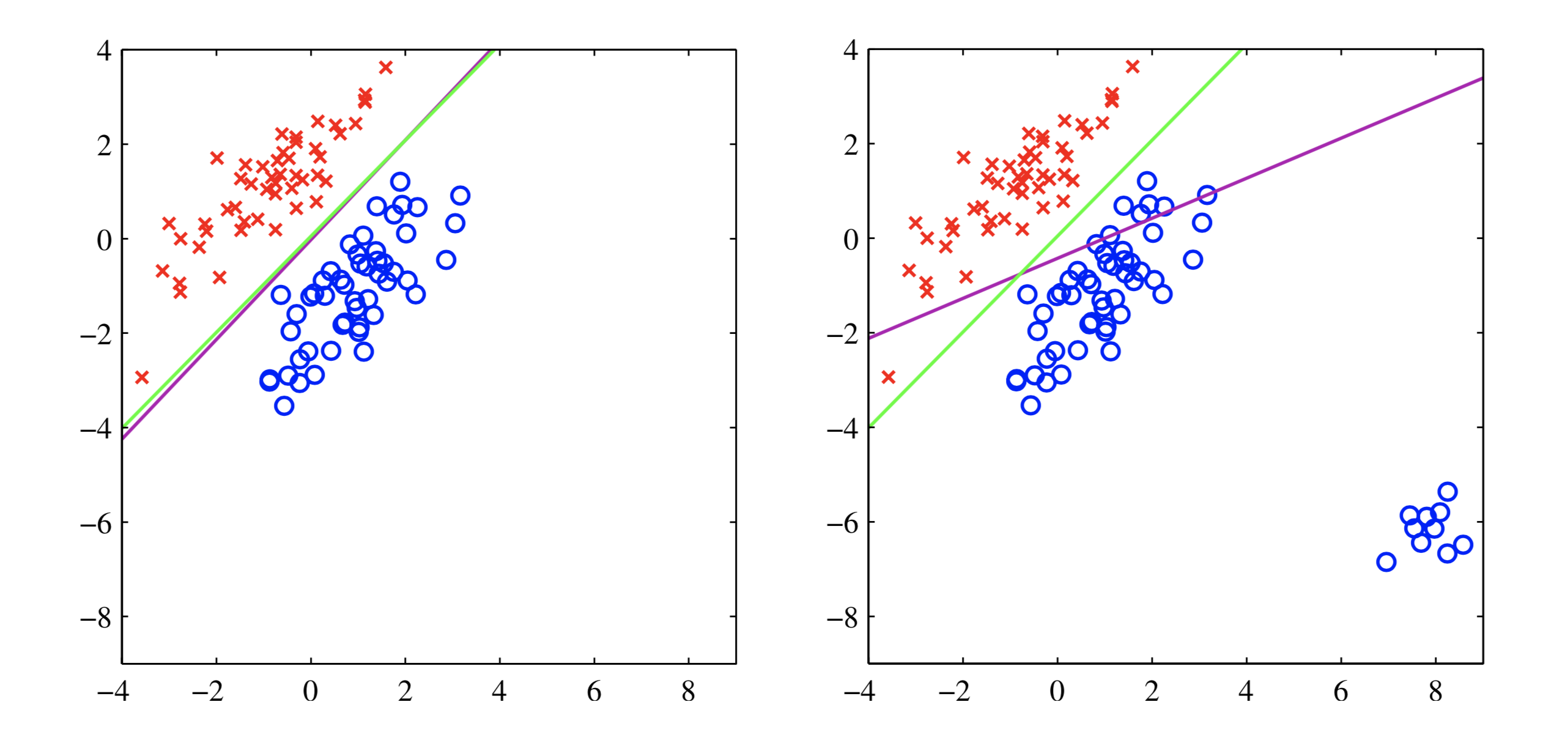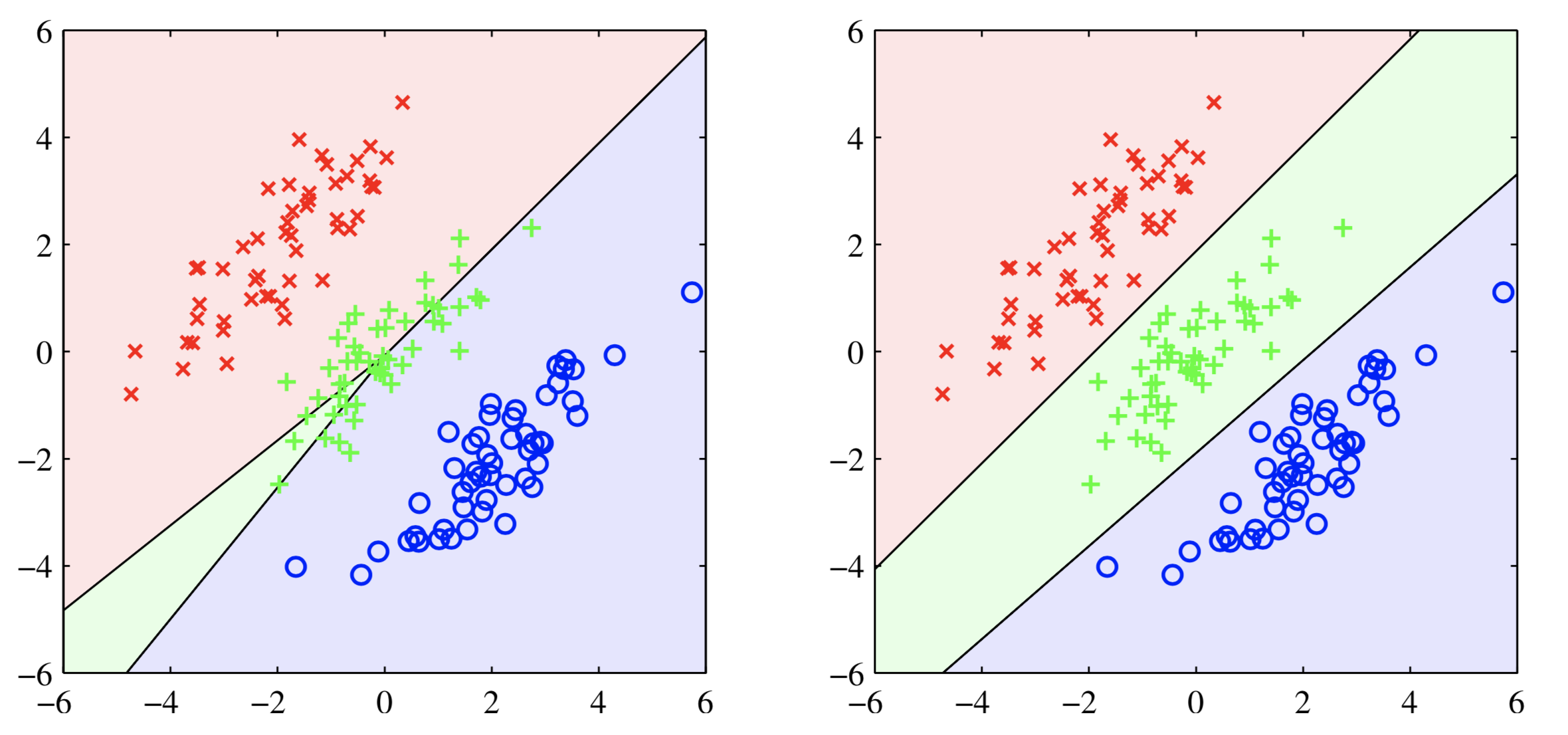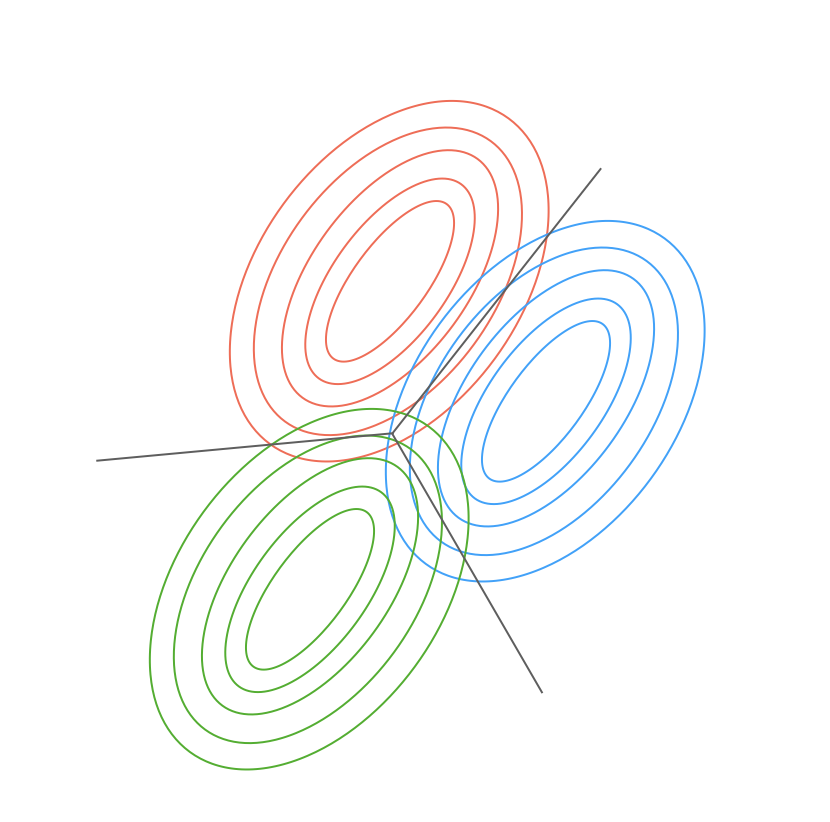## Fisher’s Linear Discriminant（LDA）

### 二分类

1. $y(x)=\mathbf{w^{T}x}$将样本$\mathbf{x}$映射到一维
2. 在一维上分类，$y(x)\geq y_0$属于正类，$y(x)<y_0$负类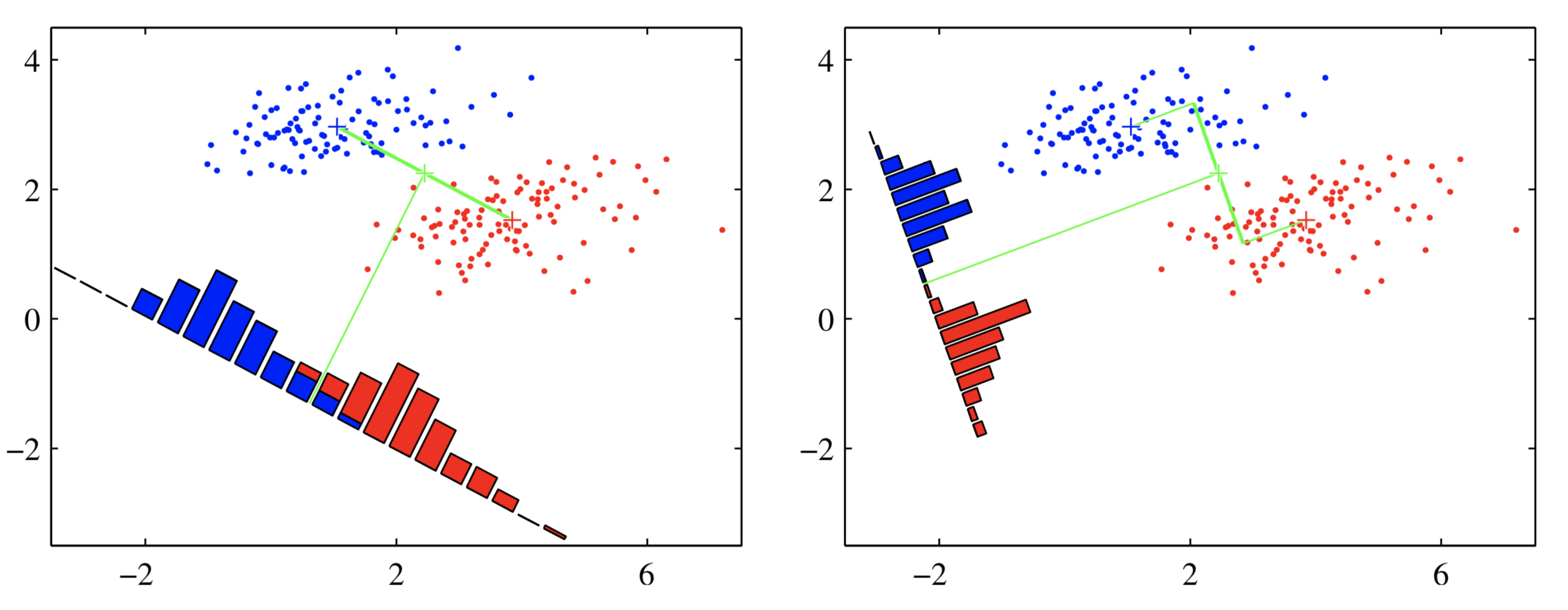$\mathbf{S_B,S_W}$定义为：

## 感知机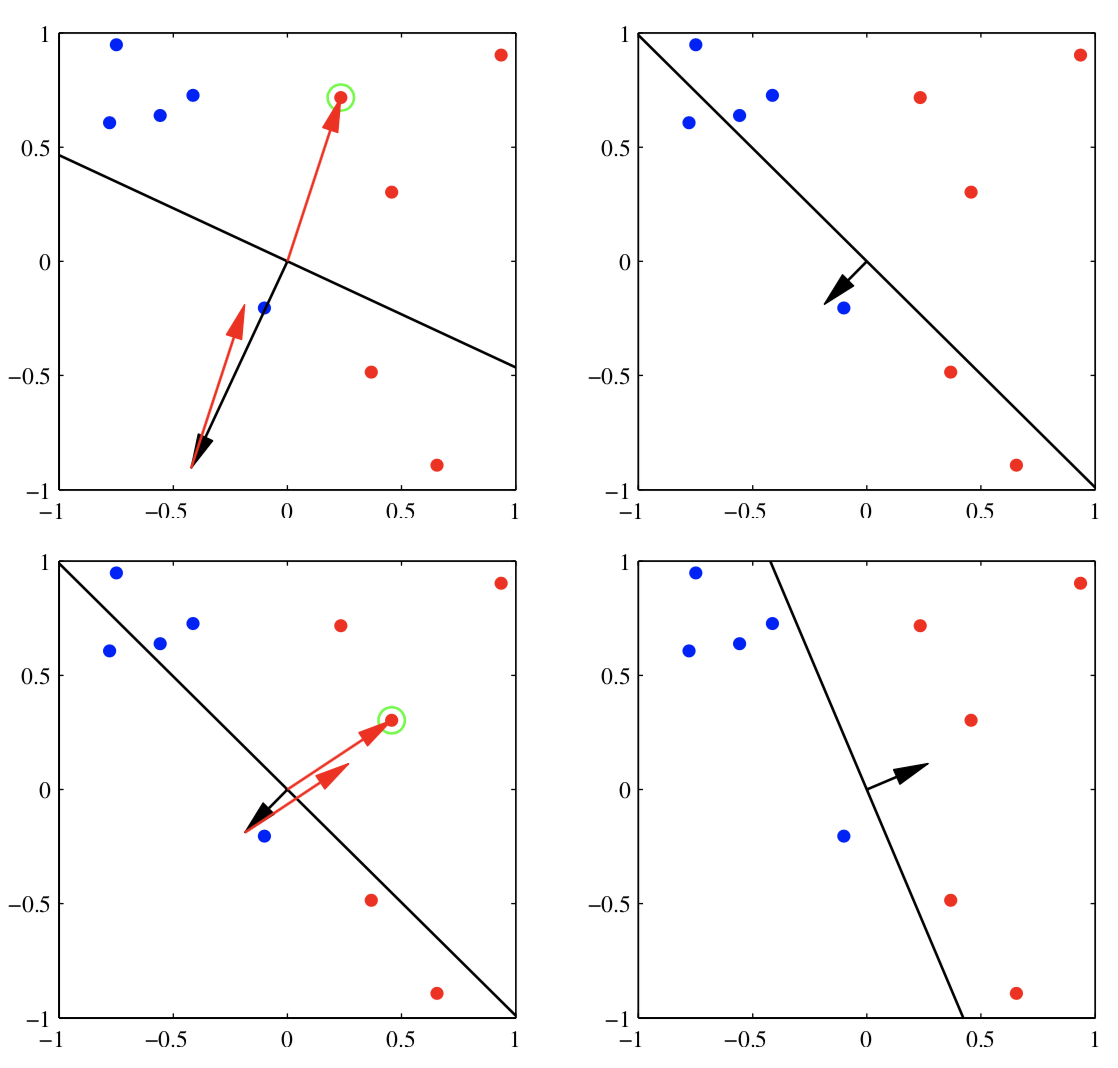1. 即使在线性可分的情况下，感知机的收敛性能够得到保证，但是可能会收敛很慢
2. 数据是否线性可分无法判断，感知机的优势其实不明显
3. 不太容易拓展到多分类情况